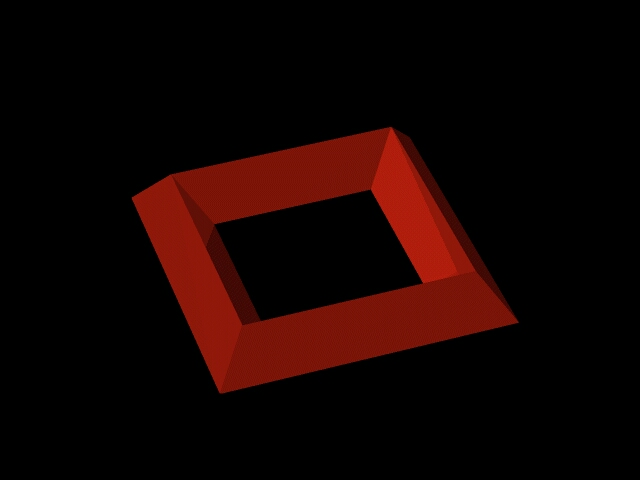A little theory:You may be asking what is this?   This program resulted from considerations based on mathematical Group Theory. The musical scale of the western hemisphere comprises of 12 semi tones. These 12 tones may be viewed as the elements of the cyclic group Z12. It can be shown that Z12 is the direct products of the groups Z3 and Z4. This is not trivial, for example, Z2 and Z6 do not yield Z12. We have been asking which musical implications this fact may have. The mathematical proof is rather simple, its interpretation in musical terms is less simple. We are not going into details, and you should not worry if you do not grasp the exposition at once. After all, it took us quite some time to sort this out. The result of our observations appeared intriguing at first sight, but eventually logical. Z3 represents the group of the three diminished chords (stacked minor thirds) and Z4 represents the four augmented chords (stacked major thirds). For the sake of completeness we would like to mention that Z2 represents the group of the whole tone scales.   Example: ·      An element of Z3 : C - D# - F# - A ·      An element of Z4 : C - E - G#   The model made with LED's should be thought of as three dimensional, the center square being the ridge of a roof. Then it should be clear that the inner and the outer square are directly adjacent. In each corner of the square there is a perpendicular triangle. The three squares represent Z3 (circumference) and the four triangles represent Z4 (dissection). No matter which note you start with constructing the model from the intervals, it will always be sound. The diagonals represent the two whole tone scales, and even the circle of fifths can be found as a regular pattern. The blue note really catches the eye. Expressed in mathematical terms, we see that the fifth, fourth, a semitone up, and a semitone down are the generators of the Z12, each of them generates the group.The groups Z3 x Z4 and = Z 4 x Z3 are isomorphic. Both can be visualized as a torus, or donut, hence the name. The first one is an outer square with triangular dissection and the second a triangle with quadratic dissection. Solid models can be crafted from wood or wire. For the program we chose the square for a smoother display on the screen, a wooden model of the triangle looks nicer in 3D. Again, group theoretically they are equivalent. Much in music can be explained using mathematics, this is just our humble contribution. Have fun with further explorations.   Hajü & Holger   Download: A little collection of formulas